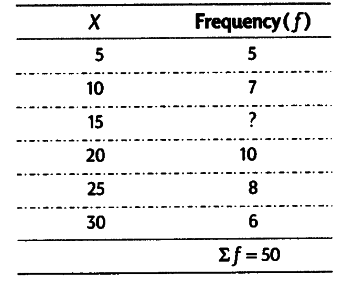# Find unknown frequency in the table given below?

Find unknown frequency in the table given below.To find unknown frequency subtract the sum of known frequencies from sum of total frequencies
Σf = 50
Sum of Known Frequency = 5+7+10+8+6 = 36
Unknown Frequency = Total Frequency - Sum of Known Frequencies= 50-36=14